# ggOceanMaps User Manual

#### 11 January, 2021

The ggOceanMaps package for R allows plotting data on bathymetric maps using ggplot2. The package is designed for ocean sciences and greatly simplifies bathymetric map plotting anywhere around the globe. ggOceanMaps uses openly available geographic data. Citing the particular data sources is advised by the CC-BY licenses whenever maps from the package are published (see the Citations and data sources section). Note that the package comes with absolutely no warranty and that maps generated by the package are meant for plotting scientific data only. The maps are coarse generalizations of third party data and therefore inaccurate.

# Basic use

ggOceanMaps extends on ggplot2. Data that contain geographic information can be plotted on the maps generated by ggOceanMaps using the ggplot2 layers separated by the `+` operator. The package uses spatial shapefiles, GIS packages for R to manipulate, and the ggspatial package to help to plot these shapefiles. The shapefile plotting is conducted internally in the `basemap` function and uses ggplot’s sf object plotting capabilities.

The primary aim of ggOceanMaps is to make plotting oceanographic spatial data as simple as feasible, but also flexible for custom modifications. The “as simple as feasible” part will be covered in this section, while the “flexible for custom modifications” part is covered in the Advanced use section. The basic use section of this tutorial assumes that the user knows how to use ggplot. If you are not familiar with this package, you may read the Data visualization section in Hadley Wickham & Garrett Grolemund. This tutorial does not describe functions in ggOceanMaps but rather focusses on how to use them. Make sure to refer to the function documentation while reading the tutorial. The package requires the `ggOceanMapsData` package which is not available on CRAN but should be installed automatically when you load `ggOceanMaps` for the first time. If the automatic installation does not work, please use:

``install.packages("ggOceanMapsData", repos = c("https://mikkovihtakari.github.io/drat", "https://cloud.r-project.org"))``

Once the packages have been installed, you can load them normally:

``````library(ggOceanMapsData)
library(ggOceanMaps)``````

## Limits

To ensure simplicity, ggOceanMaps package attempts to use decimal degree coordinate system as much as possible. This system represents coordinates on a sphere, while maps are plotted in two dimensions. Therefore, the underlying map data have to be projected using different mathematical algorithms depending on the geographic location. The simplest way of defining the geographic location is to use the `limits` argument with decimal degrees. The limits argument can be defined either as a numeric vector of length 1 or 4. Specifying the argument as a single integer between 30 and 88 or -88 and -30 plots a polar stereographic map for the Arctic or Antarctic, respectively.

``````library(ggOceanMaps)
basemap(limits = 60)``````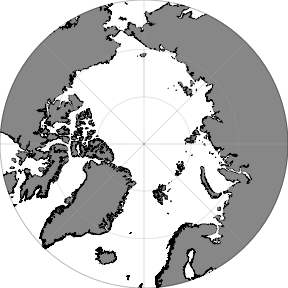Rectangular maps are plotted by specifying the `limits` argument as a numeric vector of length 4 where the first element defines the start longitude, the second element the end longitude, the third element the minimum latitude and the fourth element the maximum latitude of the bounding box:

``basemap(limits = c(-20, 20, 40, 59))``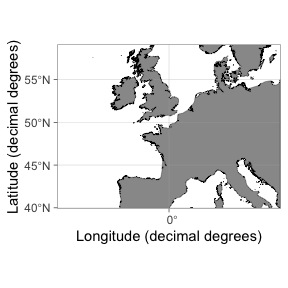Limiting maps using decimal degrees is somewhat counter-intuitive because maps plotted for polar regions (>= 60 or <= -60 latitude) are actually projected to Arctic and Antarctic polar stereographic systems. Because decimal degrees are angular units running counter-clockwise, also the longitude limits have to be defined counter-clockwise. Projected maps with decimal degree `limits` will lead to expanded limits towards the poles when using Arctic and Atlantic Polar Stereographic projections because decimal degrees represent a sphere: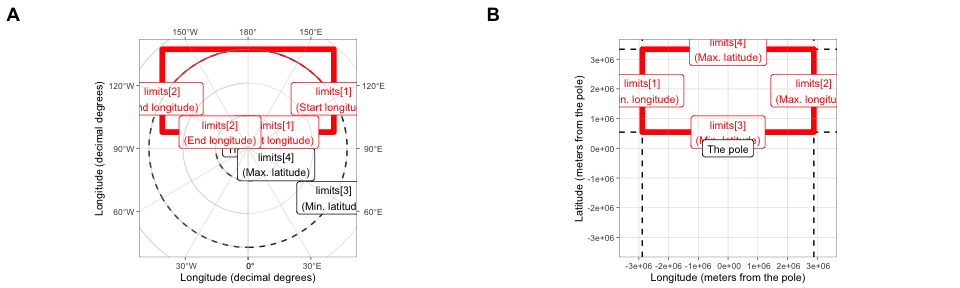The figure above: Limiting rectangular basemaps is done by placing four coordinates to the limit argument. A) If the limits are in decimal degrees, the longitude limits (`[1:2]`) specify the start and end segments of corresponding angular lines that should reside inside the map area. The longitude limits are defined counter-clockwise. The latitude limits `[3:4]` define the parallels that should reside inside the limited region given the longitude segments. Note that the resulting limited region (polygon with thick red borders) becomes wider than the polygon defined by the coordinates (thin red borders). The example limits are `c(120, -120, 60, 80)`. B) If the limits are given as projected coordinates or as decimal degrees for maps with |latitude| < 60, limits elements represent lines encompassing the map area in cartesian space. The example limits are the limits from A) projected to the Arctic stereographic (crs = 3995). When limiting basemaps using data, the limits are calculated for the maximum reach of projected coordinates as in B but with an added buffer to place all points inside the map area.

As an example:

``````dt <- data.frame(lon = c(160, 160, -160, -160), lat = c(60, 80, 80, 60))

basemap(limits = c(160, -160, 60, 80)) +
geom_spatial_polygon(data = dt, aes(x = lon, y = lat), fill = NA, color = "red")``````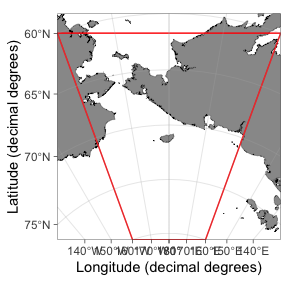Exact control of map limits can be difficult using decimal degree limits in polar regions. The `limits` argument also allows specifying the limits in the underlying projected coordinate units. First, we will need to find out how these units look like:

``basemap(limits = 60, projection.grid = TRUE, grid.col = "red")``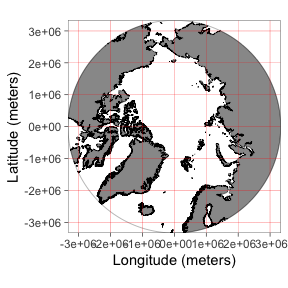The `projection.grid` argument plots a grid using the projected actual map coordinates instead of decimal degrees. The grid helps in defining the `limits` using projected coordinates giving better control over the map limits than decimal degree coordinates. The automatic shapefile definition algorithm does not work for projected coordinates. Therefore, if the limits are not given as decimal degrees (any longitude outside the range [-180, 180] or latitude [-90, 90]), the function asks to specify `shapefiles`. The `shapefiles` can be defined by partially matching the names of the pre-made shapefiles in `shapefile_list` (e.g. “Ar” would be enough for “ArcticStereographic”):

``basemap(limits = c(-2e6, 1e6, 0, 3e6), shapefiles = "Arctic") ``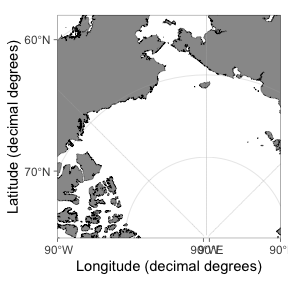## Data limits

The limits of a map can also be defined by inputting a data frame to the `data` argument. The limits are automatically defined allowing the user to quickly find limits for a desired spatial dataset:

``````dt <- expand.grid(lon = c(160, -160), lat = c(60, 80))

basemap(data = dt) +
geom_spatial_point(data = dt, aes(x = lon, y = lat), color = "red")``````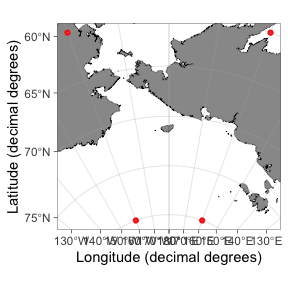Note how the function expands the map to make all data fit inside the map area compared to a similar plot using the `limits` argument above. The function automatically detects columns containing longitude and latitude information. The automatic detection algorithm is not very advanced and it is recommended to use intuitive column names for longitude (such as “lon”, “long”, or “longitude”) and latitude (“lat”, “latitude”) columns. The coordinate data have to be given as decimal degrees for the `data` argument to function.

## Bathymetry and glaciers

It is advised to limit your map first and only then plot bathymetry and glaciers to save the processing time of your computer (the bathymetry shapes can be large). Bathymetry is plotted simply by specifying `bathymetry = TRUE`:

``basemap(limits = c(100, 160, -20, 30), bathymetry = TRUE)``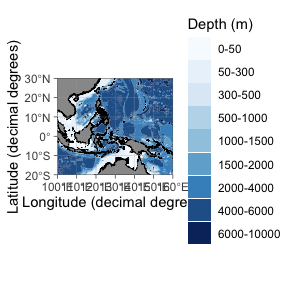Glaciers can be plotted using the `glaciers` argument:

``basemap(limits = 60, glaciers = TRUE, bathymetry = TRUE)``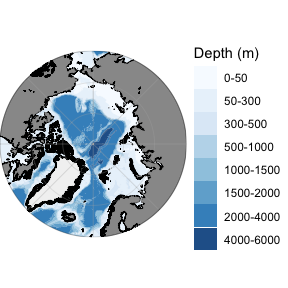## Adding data to maps

The `basemap(...)` function works almost similarly to the `ggplot(...)` function as a base for adding further layers to the plot. The difference between the `basemap()` and the `ggplot()` is that the `basemap()` plot already contains multiple ggplot layers. All layers except bathymetry have no other `aes` mapping than `x`, `y` and `group`. Bathymetry is mapped to `fill` or `color` color in addition. This means that when you add ggplot layers, you need to specify the `data` argument explicitly as shown below. Another difference is that basemaps are plotted using projected coordinates. The ggspatial and ggplot’s `geom_sf` functions convert the coordinates automatically to the projected coordinates:

``````dt <- data.frame(lon = c(seq(-180, 0, 30), seq(30, 180, 30)), lat = -70)
basemap(limits = -60, glaciers = TRUE) + geom_spatial_point(data = dt, aes(x = lon, y = lat), color = "red")``````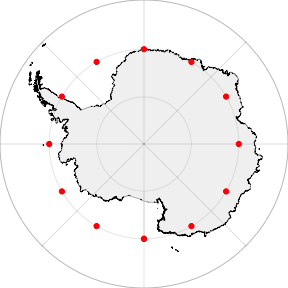The ggplot functions can also be used, but the coordinates need to be transformed to the basemap projection first using the `transform_coord` function:

``````basemap(limits = -60, glaciers = TRUE) +
geom_point(data = transform_coord(dt), aes(x = lon, y = lat), color = "red")``````Note that the maps plotted in temperate and tropical regions are not projected. Consequently, decimal degrees work for such maps directly:

``````dt <- data.frame(lon = c(-100, -80, -60), lat = c(10, 25, 40), var = c("a", "a", "b"))
basemap(data = dt) + geom_point(data = dt, aes(x = lon, y = lat), color = "red")``````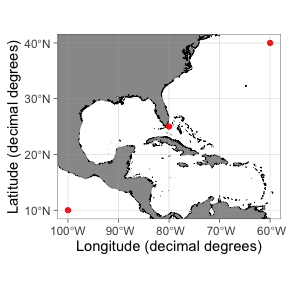The `transform_coord` function detects the projection automatically, given that the map is limited using a similar range of coordinates. Therefore you can use the `transform_coord` as demonstrated above whenever using standard ggplot layers.

``````transform_coord(data.frame(lon = -80, lat = 25), bind = TRUE)
#>   lon lat lon.proj lat.proj
#> 1 -80  25      -80       25``````

## Rotating maps

The stereographic maps can be rotated to point towards north using the `rotate` argument:

``basemap(limits = c(-160, -80, 60, 85), rotate = TRUE)``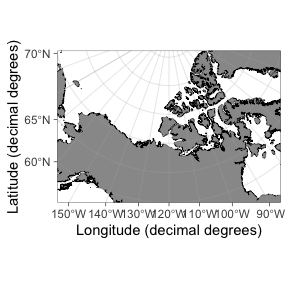A word of warning, however: the rotation involves reprojecting all shapefiles in their entirety and is memory consuming especially for large bathymetry shapes. Make sure to limit your map correctly before you add bathymetry. The rotation is still experimental and does not work for `limit`s defined using projected coordinates or `data`. This functionality is likely to contain a whole lot of bugs. Rotation does not currently work within the `transform_coord()` function. Use the `ggspatial::geom_spatial_*` functions when adding data to rotated maps.

## Quick map

The `qmap` function is designed as a shortcut to quickly take a look at a spatial dataset similar to the ggplot’s `qplot` function. This function is supposed to automatically detect the type of data fed into the function and plot a map using appropriate geometries, limits, and projection. The function has not been developed properly yet and requires user feedback and testing.

``qmap(dt, color = I("red")) # set color````qmap(dt, color = var) # map color``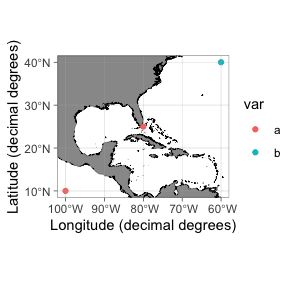# Advanced use

This section focuses on flexibility and user modifications. It is assumed that advanced users understand the basics of geographic information systems (GIS) and how to use these systems in R (e.g. see the Making Maps with R chapter in Lovelace et al. 2020).

## Projections

The `basemap` function uses the `limits` argument to automatically detect the required projection for a map (or the `data` argument to calculate `limits`). The algorithms deciding which projection to use are defined in `define_shapefiles` and `shapefile_list` functions. These conditions are expected to change during the development of the package and documented information here or in the `basemap` function might be outdated. Make sure to check the projection definition functions for up-to-date information. At the time of writing, the function uses three different projections (given as EPSG codes):

• 3995 WGS 84 / Arctic Polar Stereographic. Called “ArcticStereographic”. For max latitude (`limits`) >= 60 (if min latitude (`limits`) >= 30), and single integer latitudes >= 30 and <= 89.
• 3031 WGS 84 / Antarctic Polar Stereographic. Called “AntarcticStereographic”. For max latitude (`limits`) <= -60 (if min latitude (`limits`) <= -30), and single integer latitudes <= -30 and >= -89.
• 4326 WGS 84 / World Geodetic System 1984, used in GPS. Called “DecimalDegree”. For min latitude (`limits`) < 30 or > -30, max latitude (`limits`) < 60 or > -60, and single integer latitudes < 30 and > -30.

The `basemap` function uses pre-transformed shapefiles for each of the projections above (defined in `shapefile_list`). This is to make the plotting quicker and more memory efficient but leads to larger data size required by the package.

## Appearance

### Bathymetry styles

The `basemap` function contains four pre-made bathymetry styles defined using the `bathy.style` argument. Two of these alternatives (`"poly_*"`) have been mapped to `fill` using the `geom_polygon` function, while the two others (`contour_*`) have been mapped to `color`. The default style (`"poly_blues"`) has been displayed throughout this user manual. The other styles are:

``basemap(limits = c(0, 46, 70, 81), bathymetry = TRUE, bathy.style = "poly_greys")``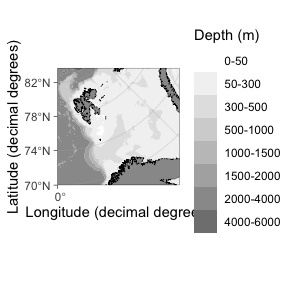``basemap(limits = c(0, 46, 70, 81), bathymetry = TRUE, bathy.style = "contour_blues")``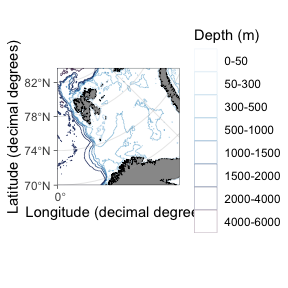``basemap(limits = c(0, 46, 70, 81), bathymetry = TRUE, bathy.style = "contour_grey")``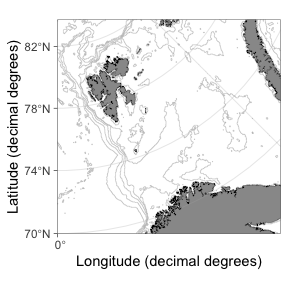#### Customizing bathymetry styles

The `bathy.style = "poly_*"` bathymetry polygons are mapped to `geom_fill_discrete` and can be modifying using standard ggplot syntax:

``basemap(limits = c(-140, -105, 20, 40), bathymetry = TRUE) + scale_fill_viridis_d("Water depth (m)")``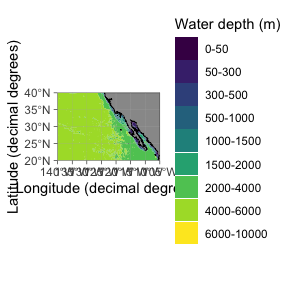The `bathy.style = "contour_*"` bathymetry lines are mapped to `geom_color_discrete` and can be modifying using standard ggplot syntax:

``basemap(limits = c(0, 60, 68, 80), bathymetry = TRUE, bathy.style = "contour_blues") + scale_color_hue()``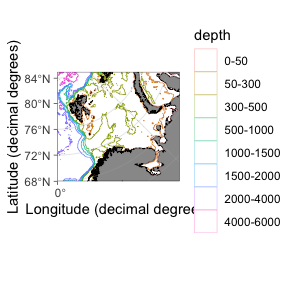### Graphical parameters

The `basemap` function uses graphical parameters that (very objectively) happen to please the eye of the author and have worked in the applications needed by the author. The default parameters may suddenly change without warning. You may want to modify the appearances of a `basemap` to your own liking. This can be done using the `*.col` (fill), `*.border.col` (line color) and `*.size` (line width) arguments:

``````basemap(limits = c(-20, 30, 55, 70), glaciers = TRUE,
bathymetry = TRUE, bathy.style = "poly_greys",
land.col = "#eeeac4", gla.col = "cadetblue",
land.border.col = NA, gla.border.col = NA,
grid.size = 0.05)``````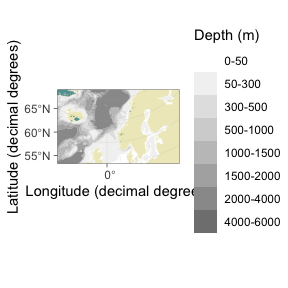Grid lines can be removed by setting the `grid.col` to `NA`. Axis labels can be manipulated using standard ggplot code:

``basemap(limits = c(124, 148, 31, 50), grid.col = NA) + labs(x = NULL, y = "Only latitude for you, ...")``